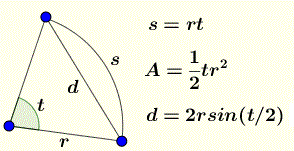# Arc Length, Chord and Area of a sector - Geometry Calculator

An easy to use online calculator to calculate the arc length s , the length d of the Chord and the area A of a sector given its radius and its central angle t.

## Formulas for arc Length, chord and area of a sectorFigure 1. formulas for arc Length, chord and area of a sector In the above formulas t is in radians. In this calculator you may enter the angle in degrees, or radians or both.

## How to use the calculator

Enter the radius and central angle in DEGREES, RADIANS or both as positive real numbers and press "calculate". The outputs are the arclength s, area A of the sector and the length d of the chord.

 radius = angle in radians = angle in degrees = for angle in radians arclength s = area A = chord d = for angle in degrees arclength = area A = chord d =